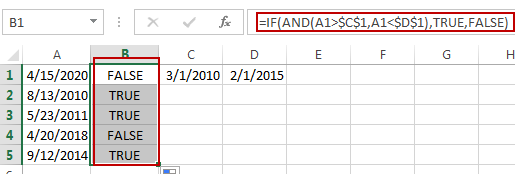# Returning Value if the Dates Fall between Two Dates

This post will guide you how to calculate value between two dates if the dates falls those two dates in Excel. How do return a value if the dates fall between two given dates in excel. How to use a formula to determine whether a date falls between two dates in Excel. How to check if a date falls between a range of two dates in Excel.

## Determine Whether a Date Falls between Two Dates

Assuming that you have a list of data that contain dates in one column or range, and you want to check those dates if fall between two given dates in range C1:D1. How to achieve it. You can create a formula based on the IF function, and the AND function. Like this:

=IF(AND(A1>\$C\$1,A1<\$D\$1),TRUE,FALSE)

Type this formula into Cell B1, and press Enter key in your keyboard, and then drag the AutoFill Handle from Cell B1 to B5.If the data falls between the given two dates, and this formula return TRUE, otherwise, return FALSE.

### Related Functions

• Excel IF function
The Excel IF function perform a logical test to return one value if the condition is TRUE and return another value if the condition is FALSE. The IF function is a build-in function in Microsoft Excel and it is categorized as a Logical Function.The syntax of the IF function is as below:= IF (condition, [true_value], [false_value])….
• Excel AND function
The Excel AND function returns TRUE if all of arguments are TRUE, and it returns FALSE if any of arguments are FALSE.The syntax of the AND function is as below:= AND (condition1,[condition2],…) …